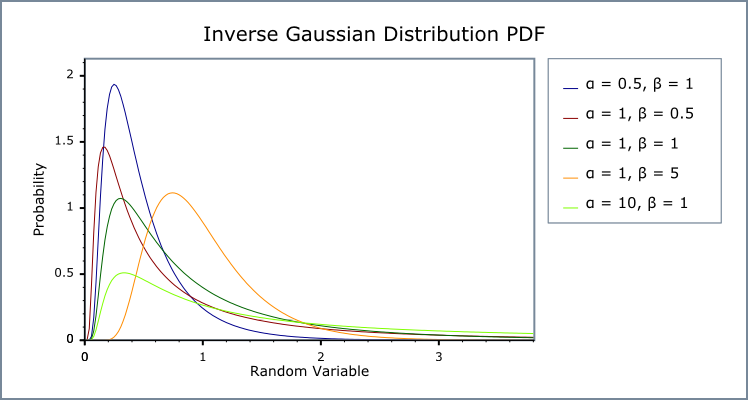#Boost C++ Libraries

...one of the most highly regarded and expertly designed C++ library projects in the world.

#### Inverse Gaussian (or Inverse Normal) Distribution

`#include <boost/math/distributions/inverse_gaussian.hpp>`
```namespace boost{ namespace math{

template <class RealType = double,
class Policy   = policies::policy<> >
class inverse_gaussian_distribution
{
public:
typedef RealType value_type;
typedef Policy   policy_type;

inverse_gaussian_distribution(RealType mean = 1, RealType scale = 1);

RealType mean()const; // mean default 1.
RealType scale()const; // Optional scale, default 1 (unscaled).
RealType shape()const; // Shape = scale/mean.
};
typedef inverse_gaussian_distribution<double> inverse_gaussian;

}} // namespace boost // namespace math
```

The Inverse Gaussian distribution distribution is a continuous probability distribution.

The distribution is also called 'normal-inverse Gaussian distribution', and 'normal Inverse' distribution.

It is also convenient to provide unity as default for both mean and scale. This is the Standard form for all distributions. The Inverse Gaussian distribution was first studied in relation to Brownian motion. In 1956 M.C.K. Tweedie used the name Inverse Gaussian because there is an inverse relationship between the time to cover a unit distance and distance covered in unit time. The inverse Gaussian is one of family of distributions that have been called the Tweedie distributions.

(So inverse in the name may mislead: it does not relate to the inverse of a distribution).

The tails of the distribution decrease more slowly than the normal distribution. It is therefore suitable to model phenomena where numerically large values are more probable than is the case for the normal distribution. For stock market returns and prices, a key characteristic is that it models that extremely large variations from typical (crashes) can occur even when almost all (normal) variations are small.

Examples are returns from financial assets and turbulent wind speeds.

The normal-inverse Gaussian distributions form a subclass of the generalised hyperbolic distributions.

If you want a `double` precision inverse_gaussian distribution you can use

`boost::math::inverse_gaussian_distribution<>`

or, more conveniently, you can write

```using boost::math::inverse_gaussian;
inverse_gaussian my_ig(2, 3);
```

For mean parameters μ and scale (also called precision) parameter λ, and random variate x, the inverse_gaussian distribution is defined by the probability density function (PDF):

f(x;μ, λ) = √(λ/2πx3) e-λ(x-μ)²/2μ²x

and Cumulative Density Function (CDF):

F(x;μ, λ) = Φ{√(λx) (xμ-1)} + e2μ/λ Φ{-√(λ/μ) (1+x/μ)}

where Φ is the standard normal distribution CDF.

The following graphs illustrate how the PDF and CDF of the inverse_gaussian distribution varies for a few values of parameters μ and λ:Tweedie also provided 3 other parameterisations where (μ and λ) are replaced by their ratio φ = λ/μ and by 1/μ: these forms may be more suitable for Bayesian applications. These can be found on Seshadri, page 2 and are also discussed by Chhikara and Folks on page 105. Another related parameterisation, the __wald_distrib (where mean μ is unity) is also provided.

##### Member Functions
```inverse_gaussian_distribution(RealType df = 1, RealType scale = 1); // optionally scaled.
```

Constructs an inverse_gaussian distribution with μ mean, and scale λ, with both default values 1.

Requires that both the mean μ parameter and scale λ are greater than zero, otherwise calls domain_error.

```RealType mean()const;
```

Returns the mean μ parameter of this distribution.

```RealType scale()const;
```

Returns the scale λ parameter of this distribution.

##### Non-member Accessors

All the usual non-member accessor functions that are generic to all distributions are supported: Cumulative Distribution Function, Probability Density Function, Quantile, Hazard Function, Cumulative Hazard Function, mean, median, mode, variance, standard deviation, skewness, kurtosis, kurtosis_excess, range and support.

The domain of the random variate is [0,+∞).

NoteUnlike some definitions, this implementation supports a random variate equal to zero as a special case, returning zero for both pdf and cdf.
##### Accuracy

The inverse_gaussian distribution is implemented in terms of the exponential function and standard normal distribution N0,1 Φ : refer to the accuracy data for those functions for more information. But in general, gamma (and thus inverse gamma) results are often accurate to a few epsilon, >14 decimal digits accuracy for 64-bit double.

##### Implementation

In the following table μ is the mean parameter and λ is the scale parameter of the inverse_gaussian distribution, x is the random variate, p is the probability and q = 1-p its complement. Parameters μ for shape and λ for scale are used for the inverse gaussian function.

Function

Implementation Notes

pdf

√(λ/ 2πx3) e-λ(x - μ)²/ 2μ²x

cdf

Φ{√(λx) (xμ-1)} + e2μ/λ Φ{-√(λ/μ) (1+x/μ)}

cdf complement

using complement of Φ above.

quantile

No closed form known. Estimated using a guess refined by Newton-Raphson iteration.

quantile from the complement

No closed form known. Estimated using a guess refined by Newton-Raphson iteration.

mode

μ {√(1+9μ²/4λ²)² - 3μ/2λ}

median

No closed form analytic equation is known, but is evaluated as quantile(0.5)

mean

μ

variance

μ³/λ

skewness

3 √ (μ/λ)

kurtosis_excess

15μ/λ

kurtosis

12μ/λ

##### References
1. Wald, A. (1947). Sequential analysis. Wiley, NY.
2. The Inverse Gaussian distribution : theory, methodology, and applications, Raj S. Chhikara, J. Leroy Folks. ISBN 0824779975 (1989).
3. The Inverse Gaussian distribution : statistical theory and applications, Seshadri, V , ISBN - 0387986189 (pbk) (Dewey 519.2) (1998).
4. Numpy and Scipy Documentation.
5. R statmod invgauss functions.
6. R SuppDists invGauss functions. (Note that these R implementations names differ in case).
7. StatSci.org invgauss help.
8. invgauss R source.
9. pwald, qwald.
10. Brighton Webs wald.
 Copyright © 2006-2010, 2012, 2013 Paul A. Bristow, Christopher Kormanyos, Hubert Holin, Bruno Lalande, John Maddock, Johan Råde, Gautam Sewani, Benjamin Sobotta, Thijs van den Berg, Daryle Walker and Xiaogang Zhang Distributed under the Boost Software License, Version 1.0. (See accompanying file LICENSE_1_0.txt or copy at http://www.boost.org/LICENSE_1_0.txt)